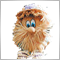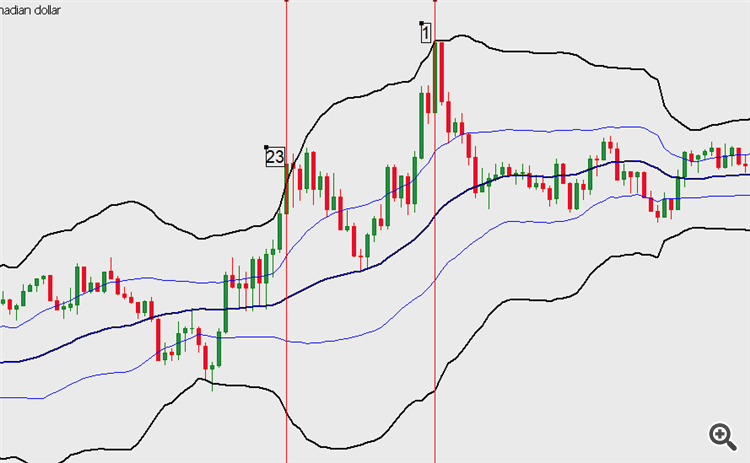24

what's wrong?  show your idea in the form of a picture

Screenshot

Files:
Screenshot_.png  19 kbModerator
270369

GeorgeReji :

Thanks for the clarifying drawing. Another question: should the intersection of the price and the iMA indicator be checked on the '0' or '1' bar?24

Thanks for the clarifying drawing. Another question: should the intersection of the price and the iMA indicator be checked on the '0' or '1' bar?

At bar 0, current bid or ask prices should equal indicator value depending on buy or sellModerator
270369

GeorgeReji :
At bar 0, current bid or ask prices should equal indicator value depending on buy or sell

I understood you. I just warn you right away: never use the "price is equal to the indicator value" condition. Use the "price crossed the indicator" condition.

The code will be a little later ...Moderator
270369

### Close a position at the intersection of the price and iMA

Code 'Close a position at the intersection of the price and iMA.mq5'

```//+------------------------------------------------------------------+
//|    Close a position at the intersection of the price and iMA.mq5 |
//+------------------------------------------------------------------+
#property version   "1.000"
/*
*/
//---
CPositionInfo  m_position;                   // object of CPositionInfo class
CSymbolInfo    m_symbol;                     // object of CSymbolInfo class

//--- input parameters
input group             "MA Fast"
input int                  Inp_MA_ma_period     = 10;          // MA: averaging period
input int                  Inp_MA_ma_shift      = 0;           // MA: horizontal shift
input ENUM_MA_METHOD       Inp_MA_ma_method     = MODE_EMA;    // MA: smoothing type
input ENUM_APPLIED_PRICE   Inp_MA_applied_price = PRICE_CLOSE; // MA: type of price
input bool     InpPrintLog          = false;       // Print log
input uchar    InpFreezeCoefficient = 1;           // Coefficient (if Freeze==0 Or StopsLevels==0)
input ulong    InpDeviation         = 10;          // Deviation, in Points (1.00045-1.00055=10 points)
input ulong    InpMagic             = 300;         // Magic number
//---
int      handle_iMA;                            // variable for storing the handle of the iMA indicator

bool     m_need_close_sell          = false;    // close all sell positions
bool     m_init_error               = false;    // error on InInit
//+------------------------------------------------------------------+
//| Expert initialization function                                   |
//+------------------------------------------------------------------+
int OnInit()
{
//--- forced initialization of variables
m_need_close_sell          = false;    // close all sell positions
m_init_error               = false;    // error on InInit
//---
ResetLastError();
if(!m_symbol.Name(Symbol())) // sets symbol name
{
Print(__FILE__," ",__FUNCTION__,", ERROR: CSymbolInfo.Name");
return(INIT_FAILED);
}
//---
//--- create handle of the indicator iMA
handle_iMA=iMA(m_symbol.Name(),Period(),Inp_MA_ma_period,Inp_MA_ma_shift,
Inp_MA_ma_method,Inp_MA_applied_price);
//--- if the handle is not created
if(handle_iMA==INVALID_HANDLE)
{
//--- tell about the failure and output the error code
PrintFormat("Failed to create handle of the iMA indicator for the symbol %s/%s, error code %d",
m_symbol.Name(),
EnumToString(Period()),
GetLastError());
//--- the indicator is stopped early
m_init_error=true;
return(INIT_SUCCEEDED);
}
//---
return(INIT_SUCCEEDED);
}
//+------------------------------------------------------------------+
//| Expert tick function                                             |
//+------------------------------------------------------------------+
void OnTick()
{
if(m_init_error)
return;
//---
{
int      count_sells          = 0;
//---
{
{
return;
}
else
}
if(m_need_close_sell)
{
if(count_sells>0)
{
ClosePositions(POSITION_TYPE_SELL);
return;
}
else
m_need_close_sell=false;
}
}
//---
double ma[];
MqlRates rates[];
ArraySetAsSeries(ma,true);
ArraySetAsSeries(rates,true);
int start_pos=0,count=3;
if(!iGetArray(handle_iMA,0,start_pos,count,ma) || CopyRates(m_symbol.Name(),Period(),start_pos,count,rates)!=count)
{
return;
}
else
{
if(rates.open<rates.close && rates.open<ma && rates.close>ma)
else
if(rates.open>rates.close && rates.open>ma && rates.close<ma)
m_need_close_sell=true;
}
//---
}
//+------------------------------------------------------------------+
//| Get value of buffers                                             |
//+------------------------------------------------------------------+
bool iGetArray(const int handle,const int buffer,const int start_pos,
const int count,double &arr_buffer[])
{
bool result=true;
if(!ArrayIsDynamic(arr_buffer))
{
if(InpPrintLog)
PrintFormat("ERROR! EA: %s, FUNCTION: %s, this a no dynamic array!",__FILE__,__FUNCTION__);
return(false);
}
ArrayFree(arr_buffer);
//--- reset error code
ResetLastError();
//--- fill a part of the iBands array with values from the indicator buffer
int copied=CopyBuffer(handle,buffer,start_pos,count,arr_buffer);
if(copied!=count)
{
//--- if the copying fails, tell the error code
if(InpPrintLog)
PrintFormat("ERROR! EA: %s, FUNCTION: %s, amount to copy: %d, copied: %d, error code %d",
__FILE__,__FUNCTION__,count,copied,GetLastError());
//--- quit with zero result - it means that the indicator is considered as not calculated
return(false);
}
return(result);
}
//+------------------------------------------------------------------+
//| Close positions                                                  |
//+------------------------------------------------------------------+
void ClosePositions(const ENUM_POSITION_TYPE pos_type)
{
for(int i=PositionsTotal()-1; i>=0; i--) // returns the number of current positions
if(m_position.SelectByIndex(i)) // selects the position by index for further access to its properties
if(m_position.Symbol()==m_symbol.Name() && m_position.Magic()==InpMagic)
if(m_position.PositionType()==pos_type)
{
{
if(!m_trade.PositionClose(m_position.Ticket())) // close a position by the specified m_symbol
if(InpPrintLog)
}
else
{
if(!m_trade.PositionClose(m_position.Ticket())) // close a position by the specified m_symbol
if(InpPrintLog)
Print(__FILE__," ",__FUNCTION__,", ERROR: ","SELL PositionClose ",m_position.Ticket(),", ",m_trade.ResultRetcodeDescription());
}
}
}
//+------------------------------------------------------------------+
//| Calculate all positions                                          |
//+------------------------------------------------------------------+
{
count_sells = 0;
for(int i=PositionsTotal()-1; i>=0; i--)
if(m_position.SelectByIndex(i)) // selects the position by index for further access to its properties
if(m_position.Symbol()==m_symbol.Name() && m_position.Magic()==InpMagic)
{
{
}
else
{
count_sells++;
}
}
}
//+------------------------------------------------------------------+
```148

I want to find out what the chart ID of a specific symbol and timeframe is, how would I go about doing that ?Moderator
270369

I want to find out what the chart ID of a specific symbol and timeframe is, how would I go about doing that ?

ChartID is a chart identifier and there is no relationship with a symbol or timeframe. If there is a graph, then it has an identifier.148

ChartID is a chart identifier and there is no relationship with a symbol or timeframe. If there is a graph, then it has an identifier.

Oh, I see3686

RZAMK:

thank you.

But you brought this code in previous pages.

Oh, maybe I didnt fully explain what I meant. I dont search the history for closed positions.

I want to find the openprice of the last position that is still open and take another position or positions according to its price.

Here you are

```//|                                                                  |
//+------------------------------------------------------------------+
double YoungestShortPositionPrice(const string symbol, const int MagicNumber) //Gewinn der Gesamtposition
{
double Price=0;
datetime time=D'01.01.1970';
//--- in allen offenen Positionen suchen

int i = PositionsTotal();
while(i-->0)
{
//--- Parameter der Order
ulong  position_ticket=PositionGetTicket(i);// das Ticket der Position
PositionSelectByTicket(position_ticket);

string position_symbol=PositionGetString(POSITION_SYMBOL); // Symbol
ulong  magic=PositionGetInteger(POSITION_MAGIC); // MagicNumber der Position
double position_lot=PositionGetDouble(POSITION_VOLUME);
ENUM_POSITION_TYPE type = (ENUM_POSITION_TYPE)PositionGetInteger(POSITION_TYPE);
datetime opentime = (datetime)PositionGetInteger(POSITION_TIME);
double position_price=PositionGetDouble(POSITION_PRICE_OPEN);

//--- wenn die MagicNumber übereinstimmt, sind Stop Loss und Take Profit nicht gesetzt
if(position_symbol==symbol
&& MagicNumber==magic
&& time < opentime
&& type == POSITION_TYPE_SELL
)
{
time=opentime;
Price = position_price;
}
}
return(Price);
}```148

I have tried to ask this on another article but it seems that no one was able to answer it. I will be using 2 bollinger bands. 2.5 and 1.0 deviations, i will check if the close of the first candle is above the 2.5 bollinger band and i have set a loop going from 5 to 50. This loop will check if any of the closes from close to close is above the 2.5 bollinger band. The part im having a hard time trying to put are these 2 conditions

1. All the candles between close and candle loop 5-50 must be above a certain bollinger band deviation.

2. Atleast one candle should be below a certain level

I'll give an example with a picture belowYou can see here close is above 2.5 bollinger band and running through the candle loop we see that close closes above 2.5 band. The blue bollinger band is a 1.0 deviation and as you can see in the picture all the candles from close to close are above lower 1.0 band and atleast one candle closes below 1.0 upper band